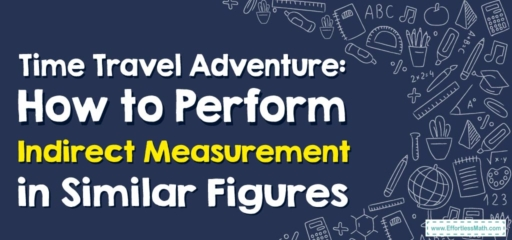# Time Travel Adventure: How to Perform Indirect Measurement in Similar Figures

Greetings, time travelers!

On our next adventure, we're journeying back to ancient times, where scientists first discovered the concept of similar figures and indirect measurement. Pack your curiosity and let's dive into the time portal!## 1. A Leap in Time: Understanding Similar Figures and Indirect Measurement

Before we set off, let’s ensure we understand the core concepts of our adventure:

• Similar Figures: These are figures that have the same shape but not necessarily the same size. They have proportional side lengths and equal angles.
• Indirect Measurement: This is a method of using properties of similar figures to measure long distances or lengths that are difficult to measure directly.

## 2. The Grand Adventure: Indirect Measurement in Similar Figures

Now that we have our time travel toolkit ready, let’s delve into the era of indirect measurements!

### Time Traveler’s Guide: Navigating Indirect Measurement in Similar Figures

#### Step 1: Recognize Similar Figures

The first step is to identify that we are dealing with similar figures. Their corresponding angles are equal, and their sides are in proportion.

#### Step 2: Set Up a Proportion

Once we’ve recognized similar figures, we set up a proportion. This involves equating ratios of corresponding sides from the two figures.

#### Step 3: Solve for the Unknown

Finally, we solve the proportion for the unknown value.

For instance, if we have two similar triangles, and the sides of the first triangle are $$2\ cm$$, $$3\ cm$$, and the sides of the second triangle are $$5\ cm$$ and $$x$$, what is the value of $$x$$?

1. Recognize Similar Figures: Both triangles are similar.
2. Set Up a Proportion: The sides are proportional, so $$\frac{2}{3} = \frac{5}{x}$$.
3. Solve for the Unknown: Cross-multiply and solve for $$x$$, giving us $$x = \frac{15}{2} = 7.5\ cm$$.

And just like that, we’ve successfully explored the world of indirect measurements in similar figures! Remember, time travelers, every era of mathematics has its unique discoveries and mysteries to unravel. Until our next historical adventure, keep your imagination alive!

### What people say about "Time Travel Adventure: How to Perform Indirect Measurement in Similar Figures - Effortless Math: We Help Students Learn to LOVE Mathematics"?

No one replied yet.

X
30% OFF

Limited time only!

Save Over 30%

SAVE $5 It was$16.99 now it is \$11.99